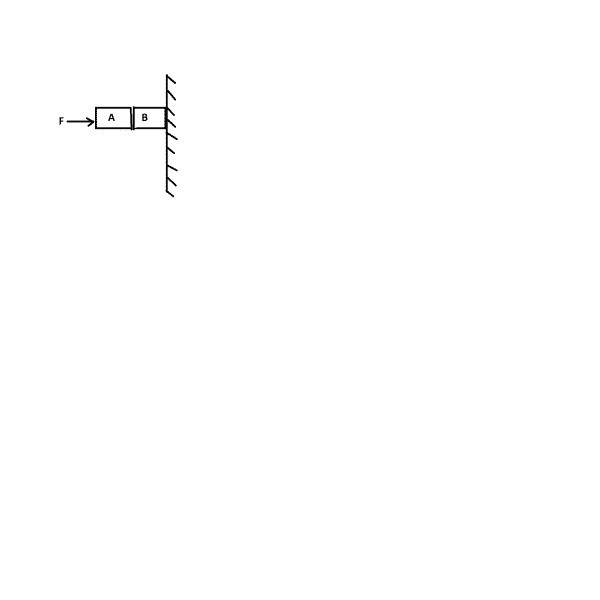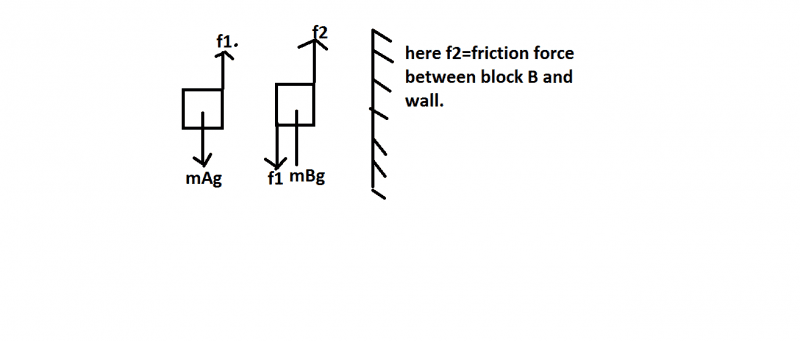# Two blocks pushed against wall

## Homework Statement

;Given in the figure are two blocks A and B of weight 20 N and 100 N, respectively. These are being pressed against a wall by a force F as shown. If the coefficient of friction between the blocks is 0.1 and between block B and the wall is 0.15, the frictional force applied by the wall on block B is[/B]

## Homework Equations

:[/B]
f 1(static friction force acting on block A)=20 N=mass of A multiplied by g.

## The Attempt at a Solution

:Here f 2=f1+ m g B= 120 N
The only thing I don't understand is why f1 is acting on block B?[/B]

haruspex
Science Advisor
Homework Helper
Gold Member
2020 Award

## Homework Statement

;Given in the figure are two blocks A and B of weight 20 N and 100 N, respectively. These are being pressed against a wall by a force F as shown. If the coefficient of friction between the blocks is 0.1 and between block B and the wall is 0.15, the frictional force applied by the wall on block B is
View attachment 81959 [/B]

## Homework Equations

:[/B]
f 1(static friction force acting on block A)=20 N=mass of A multiplied by g.

## The Attempt at a Solution

:View attachment 81960
Here f 2=f1+ m g B= 120 N
The only thing I don't understand is why f1 is acting on block B?[/B]
Because action and reaction are equal and opposite. If B is exerting a force f1 up on A, A must be exerting a force of the same magnitude down on B.

jbriggs444
Science Advisor
Homework Helper
The coefficient of [static] friction is a limit on how high the frictional force can go before the objects that are in contact start slipping. It may or may not be the applicable limit in this case. First one needs to decide whether the blocks are sliding or are sticking.

A coefficient of friction [static or dynamic] relates the tangential force of friction to the perpendicular force with which the surfaces are pressing on one another. This perpendicular force is usually called the "normal force". The word "normal" in this case means "perpendicular", not "ordinary".

With this in mind, what is the normal force between block B and the wall?

The word "normal" in this case means "perpendicular", not "ordinary".
Oh,this I know of course.Because action and reaction are equal and opposite. If B is exerting a force f1 up on A, A must be exerting a force of the same magnitude down on B.
But ,f1 is not exerted by A alone,it's a force of friction.It is due to contact between both A and B.Newton's third law will be applicable here also?

haruspex
Science Advisor
Homework Helper
Gold Member
2020 Award
But ,f1 is not exerted by A alone,it's a friction force.It is due to contact between both A and B.Newton's third law will be applicable here also?
As far as A is concerned, it is just an external force exerted on it. Doesn't matter whether it's friction or, or a nail, or magnetic...
Similarly for B. Yes the action and reaction law applies.

•gracy
Yes the action and reaction law applies.
Oh,this I didn't know of course.Thanks jbrigs444 and haruspex .

Then f 1(static friction force acting on block A) should also be =f2 +mA multiplied by g

Then f 1(static friction force acting on block A) should also be =f2 +mA multiplied by g
But if it is true we will not get the same answer for f2 i.e 120N.

jbriggs444
Science Advisor
Homework Helper
Then f 1(static friction force acting on block A) should also be =f2 +mA multiplied by g
Why?

For the same reason i.e Newton's third law.

jbriggs444
Science Advisor
Homework Helper
For the same reason i.e Newton's third law.

Show your work. Why does Newton's third law require that f2 = f1 + mAg?

Because of newton's third law f2 will also act on block A But in downward direction because f2 is acting on B upward.So for equilibrium of block A.
So f1=f2+mA g

jbriggs444
Science Advisor
Homework Helper
Because of newton's third law f2 will also act on block A But in downward direction because f2 is acting on B upward.So for equilibrium of block A.
So f1=f2+mA g
That is a completely incorrect understanding of Newton's third law. F2 acts between block B and on the wall. It does not act on block A at all.

F2 acts between block B and on the wall. It does not act on block A at all.
Oh,sorry.I misunderstood f2.My bad.I thought f2 is force of friction between the two blocks experienced by Block A.
I labeled that f2 =friction between wall and block B but forgot.Really sorry.

Last edited:
.I thought f2 is force of friction between the two blocks experienced by Block A.
Even this is wrong.But now I am clear .I know force of friction between the two blocks experienced by Block A is f1 acting in downward direction.

My ideas and thoughts are all jumbling.I think,I should sleep now.